# How does a stub filter work?

Introduction

1) The traditional approach of understanding a stub filter is very simple and is based on the assumption that the impedance of a a totally mismatched transmission line changes from short to open or open to short every quarter wave. As the stub filter is itself a transmission line, it follows that if the end of the stub filter is open, then there will be a short where the stub filter joins the main transmission line when the phase shift of the stub is 90° in one direction. In the article Understanding Coaxial RF Transmission Lines by Measurement and Calculation it was shown that the impedance of a totally mismatched transmission line remains constant along it's length and that the theory that the impedance varies every quarter wave is wrong. If the varying impedance theory is wrong, then how does a stub filter work?

Figure 1 shows a stub filter connected to a coaxial cable (transmission line) that feeds a 50 Ohm load from a 1 Volt peak (2Vp.p) Generator. This study will use the calculation of peak voltages and currents present at phase intervals of 180 degrees to establish the progressive events in the circuit and then make measurements to prove the principal of the calculations. In the measurements, the frequency of the generator is set so the the stub filter is a quarter wave (90 degrees) and the end of the stub filter is left open.

Figure 1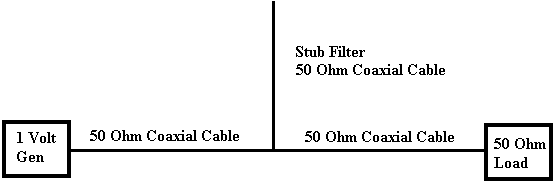It is difficult to visualise the behaviour of the signals in relation to time, so a series of figures have been made that give the instantaneous voltages and currents present for every 180 degrees phase relative to the T junction formed by the stub filter and the transmission line. The size of the T junction is taken to be so small as to have no phase shift across it and therefore no delay. The analysis becomes complex and tedious after a few revolutions of phase so only the first three peaks are analysed in order to show the principle and trends. These are followed by some measurements to confirm the basic analysis method.

Calculation of Incident and Reflected Voltages

2) An incident (forward) signal from the 1 Volt Generator arriving at the T junction formed by the stub filter joining the transmission line will be presented with two 50 Ohm cables in parallel (R = 25 Ohms). This is a mismatch and will produce a reflected signal, the magnitude of which can be found from the Reflection Coefficient.

If we ignore any reactive component, then the Reflection Coefficient = (R - Zo) / (R + Zo)

= (25 - 50)/(25 + 50) = -0.3333

The negative sign indicating that the reflected signal has changed phase by 180°.

As the incident (forward) voltage is 1 Volt, the reflected voltage will be 1 x -0.333 = -0.3333 Volts.

The reflected power will be V2 / Zo = (-0.333 x -0.333) / 50 = 0.002222 Watts

The forward power splits at the T junction and as the two cable have an impedance of (50 Ohms), half of the power will go into the stub and the other half will go on to the 50 Ohm load.

As the total output power from the generator is V2 / Zo = 12 / 50 = 0.02 Watts and the reflected power is 0.002222 Watts, the power going into the stub or on to the 50 Ohm load will be,

(0.02 - 0.002222) / 2 = 0.008888 Watts

This will produce a voltage of (W x R) ^0.5 = (0.008888 x 50) ^0.5  = 0.666 Volts

As the Transmission Coefficient is = Transmitted Voltage / Incident Voltage and the incident voltage is 1 Volt, the Transmission Coefficient for each Transmitted signal = 0.666 / 1 = 0.666

State at zero degrees

3) Assume that an incident signal has been applied at a time for the first positive peak voltage to arrive at the T junction, that this incident signal phase at the T junction is zero degrees reference, there are no losses and there are no previous signals to consider, then from the calculations above, voltages present at the junction would appear as in Figure 2.

Figure 2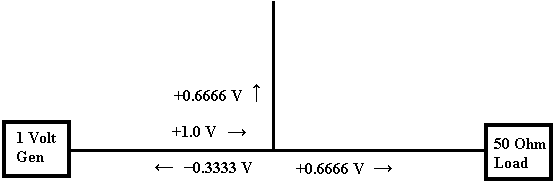When the signals reach the 180° state the signals start to add and subtract which makes the calculations lengthy using voltage, so they will be changed to current which gives simple addition and subtraction calculations. They will be changed back to voltage at the end of the calculations, so that they can be compared to the oscilloscope display in the measurement section. The currents at zero degrees phase will be as in Figure 3.

Figure 3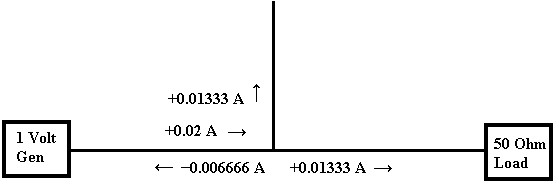These currents were simply calculated from I = V / R

At first glance the currents do not look correct because it appears that more current flowing from the junction than is passing into it and a sanity check can be made on this by calculating the power from W = V x A as per Figure 4

Figure 4The total power then becomes 0.00888 + 0.00888 + 0.00222 =  0.02 Watts

Note that the reflected power is calculated from W = V x I = -0.3333 x -0.00666 and therefore becomes positive 0.00222 Watts! The totals do sum correctly and obey Kirchhoff's law for the sum of currents at a junction but the phase change giving negative results in the reflected direction does have to be taken into account.

State at 180 degrees

4) When the signal at the T junction has reached 180 degrees, the incident current will now be at the negative peak and the currents due to this incident wave at 180 degrees will be as per Figure 5.

Figure 5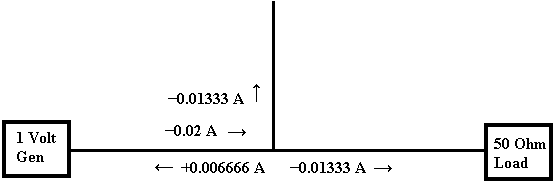The previous current at zero degrees as shown in Figure 3 above will now have travelled to the end of the stub filter and back, a total of 180 degrees. There will be no phase change at the OPEN at the end of the stub filter unlike the reflected signal phase change from the T junction. Those familiar with using a Network Analyser will know that a SHORT changes the phase of the reflected signal 180° and an OPEN does not change the phase. It can easily be deduced that a signal reflected from the stub and returning towards the T junction will see a mismatch at the T junction and will also have a Reflection Coefficient of -0.333 and a Transmission Coefficient of 0.6666 similar to the conditions for the original signal from the generator to the T junction. This will give currents for the zero degree signal as per Figure 6.

Figure 6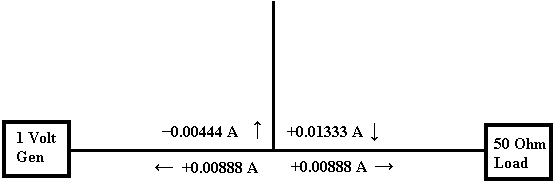The resulting sum currents of these two signals at the 180° instant (Figure 5 and Figure 6) will be as per Figure 7 which has the sums calculated with the phases taken into account.

Figure 7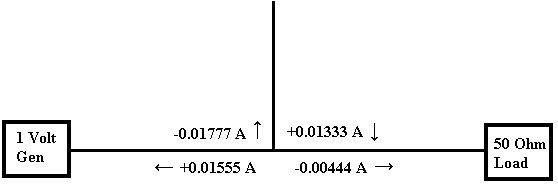It can be seen that the current reflected towards the generator has increased while the current towards the load has decreased. So far the concept is fairly simple, but on each 180 degree phase shift an extra calculation is added. One more change of phase will be shown to show the way that the reflected current and stub currents continue to build up while the current to the 50 Ohm load gradually reduces.

1  2  3  4  5  >  Page

Technical Article Index